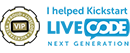## drawing lines with various length

Moderators: Klaus, FourthWorld, heatherlaine, kevinmiller

Nobbi
Posts: 2
Joined: Fri Dec 07, 2018 3:18 pm

### drawing lines with various length

I want to build a "Koch-Curve", but I don't find a possibilty to change the direction and the length of a line within the code. Who can give me a hint ?
Thanks Nobbi

bogs
Posts: 4790
Joined: Sat Feb 25, 2017 10:45 pm

### Re: drawing lines with various length

You couldn't do that with the line, you would have to use something like the freehand polygon tool instead, where you'd set the points until you eventually reach the koch snowflake level.[-hh]
VIP Livecode Opensource BackerPosts: 2262
Joined: Thu Feb 28, 2013 11:52 pm
Location: Göttingen, DE

### Re: drawing lines with various length

You have to use a graphic of style "Polygon" that displays your Koch curve.

The following function gives you the point that has length L and angle A from (0,0).
It is the core for building shapes like a Koch curve.

Code: Select all

``````local pi0=0.017453293 -- = pi/180

-- returns point with angle A and length L from (0,0)
-- angle is counterclockwise starting from 12 o'clock
-- negative angles are clockwise starting from 12 o'clock
function pointFromAngleAndLength A,L
put (90+A)*pi0 into a0
return L*cos(a0), -L*sin(a0)
end pointFromAngleAndLength``````
Here is a sample how to apply this (draws a 12-gon):

Code: Select all

``````on mouseUp
if there is no grc "poly" then
create grc "poly"
set style of grc "poly"to "polygon"
end if
put 0 into A -- starting angle
put 50 into L -- length
put (100,200) into p1 -- starting point
put p1 into p
put -30 into D -- = 30 degress clockwise (to get a 12-gon)
repeat with i=1 to abs(360 div d)
put pointFromAngleAndLength(A,L) into p2
add item 1 of p2 to item 1 of p1
add item 2 of p2 to item 2 of p1
put cr & p1 after p
end repeat
set points of grc "poly" to p
if there is no grc "dot" then
create grc "dot"
set style of grc "dot" to "oval"
set width of grc "dot" to 10
set height of grc "dot" to 10
set backcolor of grc "dot" to "255,0,0"
set opaque of grc "dot" to true
set linesize of grc "dot" to 0
end if
move grc "dot" to the points of grc "poly" in 2.0 seconds
end mouseUp``````

Now drawing a Koch curve is a (recursive) matter of math. You'll come fast to the LC lines you need if you start with exercising to draw (parts of) different n-gons.
If your length segments becomes too small there may arise numeric problems (and thus display problems) from the fact that LiveCode converts the coordinates of your computed points to integers.
[Nevertheless we should always set the not-rounded points as above to keep the overall rounding errors small.]
Have fun!
shiftLock happens

Nobbi
Posts: 2
Joined: Fri Dec 07, 2018 3:18 pm

### Re: drawing lines with various length

Hello -HH, many thanks for your example. I have tried to modify it and developed the "Initiator" and the "Generator for the Koch-Curve. I understand the structur of a recursiv function, but I don't know, how to verify it in LC. Have I to use a "function var1,var2" or a "command zeichne winkel,laenge" ? The last item shows nothing.
Nobbi
(H&H is an important software-lab in Göttingen, still existing ?)

[-hh]
VIP Livecode Opensource BackerPosts: 2262
Joined: Thu Feb 28, 2013 11:52 pm
Location: Göttingen, DE

### Re: drawing lines with various length

The KochCurve is due to integer rounding of the points more exactly drawn (less rounding error) if you use the following routine (instead of "turtle" like walking by length and angle):

Divides the line from point p1 to point p2 into a "Koch"-segments (see the attached images):

Code: Select all

``````-- p1 is listed by the previous drawStep
function drawStep p1,p2
put item 1 of p1 into u1
put item 2 of p1 into v1
put item 1 of p2 into u2
put item 2 of p2 into v2
put (u2 - u1)/3 + u1 into x1
put (v2 - v1)/3 + v1 into y1
put 2*(u2 - u1)/3 + u1 into x2
put 2*(v2 - v1)/3 + v1 into y2
put ((x1 + x2) + sqrt(3)*(y2 - y1))/2 into x3
put ((y1 + y2) + sqrt(3)*(x1 - x2))/2 into y3
return (x1,y1) &cr& (x3,y3) &cr& (x2,y2) &cr& p2
end drawStep
``````
Now iterate this for all line segements of a polygon and you get
• the Koch curve when starting from a horizontal line
• the Koch snowflake when starting from a regular (equilateral) triangle

Code: Select all

``````local g="kochcurve"
on iterate j,m
put the points of grc g into p
put the num of lines of p into pn
put line 1 of p into p1
put p1 into p0
repeat with i=2 to pn
put line i of p into p2
put cr & drawstep(p1,p2) after p0
put p2 into p1
end repeat
set points of grc g to p0
if j <= m then send "iterate j,m" to me in 1.0 seconds -- animates
end iterate
``````
Find attached a sample stack that does the above.
Note that we can have at most 7 iterations because either the length of the segments becomes too small or the number of points grows to more than 65535 (which is the max num of vertices a LC-polygon can have).

As an exercise you could do the same with adding points by angle and length (you only have to modify the drawstep but it needs more scripting).

[p.s. I'm not related to H&H]

Attachments
KochCurve.livecode.zip
Koch-Curve and Koch-Snowflake